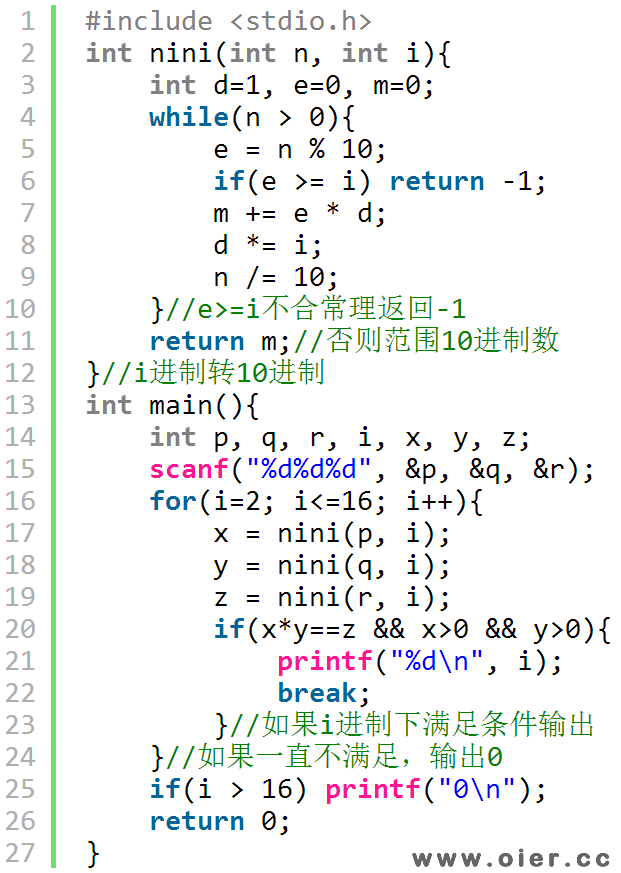NOI2.1-1973确定进制
3433+

## 题目描述

6*9 = 42 对于十进制来说是错误的，但是对于13进制来说是正确的。即, 6(13) * 9(13) = 42(13)， 而 42(13) = 4 * 131+ 2 * 130= 54(10)。 你的任务是写一段程序读入三个整数p、q和 r，然后确定一个进制 B(2<=B<=16) 使得 p * q = r. 如果 B有很多选择, 输出最小的一个。例如： p = 11, q = 11, r = 121. 则有 11(3) * 11(3) = 121(3) 因为 11(3) = 1 * 31 + 1 * 30 = 4(10) 和 121(3) = 1 * 32 + 2 * 31 + 1 * 30 = 16(10)。 对于进制 10,有 11(10) * 11(10) = 121(10)。这种情况下，应该输出 3。如果没有合适的进制，则输出 0。

## 样例输入

6 9 42

## 样例输出

13


# 程序实现